RESEARCH INTERESTS   The Concept and Results Introduction Louis de Broglie used to say that the fundamentals of physics should be reconsidered from time to time. Paul A. M. Dirac in one of his last works (1984) noted: Physics should be based on strict mathematics because the fundamental ideas of the existing theory are wrong; a new mathematical basis is needed. And since the mid-1980s, in line with those pronouncements, I began to focus my main interests on the study of fundamental physics in pure geometrical terms. I try to show how the physical notions, rules and laws would appear from a mathematical space. I carry out my research on the construction of quantum mechanics in real space, on the fusion of submicroscopic quantum mechanics with quantum gravity, and on the microstructure of the universe. I have examined how the main notions of fundamental physics (particle, mass, velocity, de Broglie wavelength, Planck's constant, Compton wavelength, etc.) can be deduced from the discrete geometry of space and the submicroscopic mechanics developed on the scale about 10-30 m (according to the modern knowledge at this size all types of interactions come together). Specifically, in collaboration with Professor Michel Bounias we worked together on the project "On the theory of space". Leading French mathematician Michel Bounias, a man of big heart, passed away on 23 March 2003. Let he be remebered forever. Here is my article written in his memory (pdf file) We started from topology, set theory and fractal geometry. In our opinion such notion as space-time is only "ad hoc" hypothesis, which is in severe conflict with such fundamental notion as a mathematical space. Time has been considered as something that independent from space but it should appear in space if one endows space by special properties and rules. A mathematical space that Professor Bounias and I have been constructed, is endowed with all the properties needed for fundamental physics. This is an antipode to the notion of space-time that cannot be called microscopic. Space structure, matter, motion and gravity The necessary and sufficient conditions allowing a previously unknown space to be explored through some 'scanning' are re-examined with respect to the measure theory by Michel Bounias and me in Refs. [16-20, 32]. In line with our theory of space, the real physical space is considered as a mathematical lattice of closely packed topological balls with the size around the Planck one, ~ 10-35 m. We have proved that such lattice is a fractal lattice and it is also manifests tessellation properties. That is why it has been called the tessellattice. In the tessellattice, a ball allows both volumetric and surface fractalities, which are associated with such fundamental physical concepts as mass and charge, or electromagnetism in general, respectively (see papers [19,22]). Note also that a valuable support of our viewpoint on the fundamentals is available in ordinary studies on fractal geometry. For instance, Jens Feder notes that a curve can be measured by means of the number of balls that cover it [J. Feder, Fractals, Plenum Press, New York and London, 1988, Ch. 2]. This in fact is a first crude step to our concept presented in works [16-20]. Thus, the tessellattice allows us to completely replace such uncertain notions as "physical vacuum", "space-time" and "aether" and hence we are able to make clear the science behind the so-called first principles sustained by orthodox quantum theories.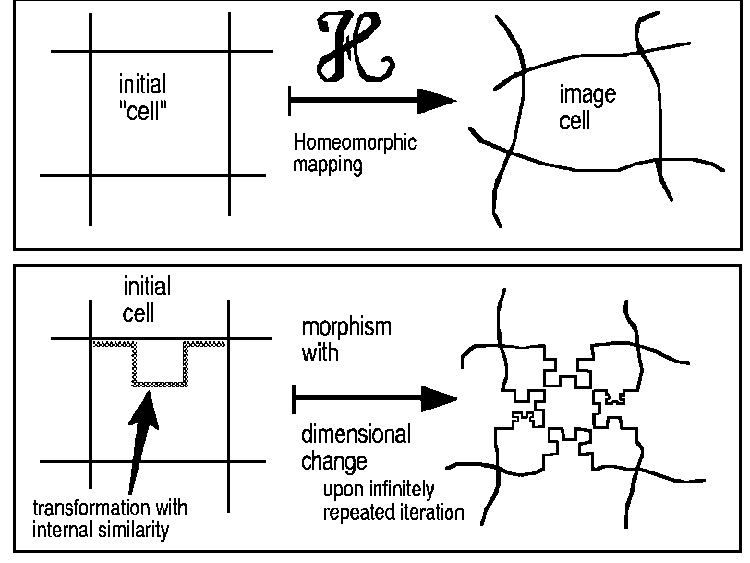Figure 1. The continuity of homeomorphic mappings of structures is broken if once a deformation involves an iterated transformation with internal self-similarity, which involves a change in the dimension of the mapped structure. A stable local deformation of the tessellattice, i.e. its deformed cell, is treated as a canonical particle. A submicroscopic mechanics of the motion of a particle in the tessellattice developed in a series of works [1-13] (or see review articles [7,10]) has a remarkable comparison with conventional quantum mechanics, which, as well-known, operates in an abstract phase space. Submicroscopic mechanics is developed in the real space and explicates an inner sub structure of the ψ-wave function giving expressions to an interlink of the particle's de Broglie wavelength λ with the range of space Λ covered by spatial excitations that accompany the moving particle. These excitations were called inertons due to the fact that they represent carriers of the force of inertia, i.e. a resistance that emerges on the side of space when a material object is moving through the space substrate, which in our theory is treated as the tessellattice. The concept allows the determination of mass as such: the mass is treated as a local deformation of the tessellattice, i.e. fractal volumetric deformation of a cell of the tessellatticce is associated with the physical notion of mass .Figure 2. Topological ball (cell, or superparticle) is represented as a triangle, figuring 3 dimensions, in a metaphorical form. A degenerate ball keeps the same dimension, in contrast with a particled ball endowed with a fractal substructure. A complete decomposition into one single ball (k = 1) conserves the volume without keeping the fractal dimension. The von Koch-like fractal has been simplified to 3 iterates for clarity. A moving particle surrounded by its inerton cloud bears a strong resemblance to a spindle; inertons move away from the particle and reach the distance λ, i.e.amplitude of the inerton cloud [1,2,4]                       Λ = λ c / v in the transversal direction to the particle's path where λ is the de Broglie wavelength of the particle and v its velocity, c is the velocity of light. Submicroscopic mechanics, which is deterministic, operates on the scale 10-30 m, or the Planck size 10-35 m. Papers [6,13,23] discuss conceptual difficulties of contemporary quantum theory and propose a way of its improvement. In particular, the principle of uncertainty                       [ x , p ]   >   h does not look as true in submicroscopic mechanics, because the momentum p is decomposed to the mass m and the velocity v; and each of these parameters is characterized by its own behavior along the particle's de Broglie wavelength >λ: the particle velocity changes from v to 0 and then is reistated to v (Refs. [1,2,4]), that is, it is not constant at all; the particle mass m also varies owing to the emission and re-absorption of inertons that transfer bits of the total particle mass (Refs. [18,32,33]). Thus, it is the inerton field that forms the specific quantum mechanical formalism at the atom size, 10-10 m (the Schroedinger and Dirac formalisms, Refs. [1,2] and , respectively). A deeper inspection of the phase transition in a quantum system when one should pass from the nonrelativistic formalism to the relativistic one has been conducted in Ref. . The examination  shows that the gravitation phenomenon to be caused by the dynamic inerton field rather than the static geometry of empty spacetime that the theory of general relativity orders. This means, in particular, that hypothetical gravitons of general relativity are merely a methodological error since the relativity does not take into account the presence of the matter waves whose subsructure is just inertons. The results obtained display: mythical gravitons have made a way for real inertons.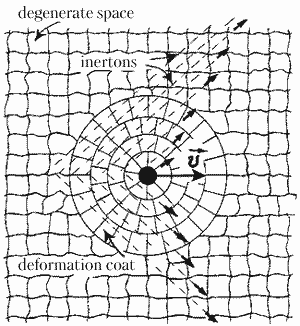Figure 3. Space net (i.e. the tessellattice) that is made up of cells, or superparticles and the motion of a particle in it. In papers [12,21,24,33] the exchange dynamics of a moving particle, i.e. exchanges with the tessellattice by fragments of the particle's fractal volumetric deformations carrying by inertons have been studied in detail. Such a mass/fractal dynamics allows the study in the framework of a specific Lagrangian and the corresponding Euler-Lagrange equations. The result shows that inertons scatter from the particle as a standing spherical wave. The deformation coat made up around the particle in the degenerate space (see Figure 3) plays the key role in the processes of emittion and the following re-absorption of inertons . It seems reasonably to say that the main idea of the research conducted in Refs. [12,21,24,33] may briefly be stated in the words: no motion, no gravity . An analysis carried out in paper  additionally allows us to estimate the velocity of inertons:   vinerton = 92 c   where   c   is the velocity of light. The inerton standing sperical wave 1/r is developing around the object studied just with this inerton velocity. But in time longer than the time concerened with the inerton dynamics we can talk about a special deformation relief around the object and this relief exactly coincides with Newton's graviational potential G M / r  [12,33]. Since laboratory methods of the study of gravity are mainly optical, we perceive Newton's graviational potential as static, though it is dynamic by its nature! A detailed analysis  enables us to conclude that the inerton field is also responsible for the nucleus stability, i.e. the inerton field generated at the scale from 10-35 to 10-30 m also acts on the size 10-13 m. The same should take place in the case of hadrons: the inerton field may be identified with gluons of quantum chromodynamics that acts at ~10-18 m. The notion of the particle spin has been successfully interpreted in Ref. . An actual canonical particle can possess the spin projection to the z-axis equal only to +1/2 or -1/2. Particles with an integer spin (0, 1, 2, ...) are compound particles.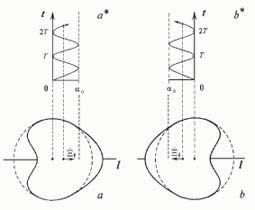Figure 4. Proper oscillations of the particle in the real space is associated with the notion of its spin. In principle such oscillations can occur not only along/against the particle path but also to the left/right. In such a manner the proposed line of research develops the model of real space, the mechanics of motion of particles, the submicroscopic theory of gravity and discloses the inner reasons of elementary interactions. Only two fundamental fields are presented in the theory: the inerton field and the photon one called also the electromagnetic field. The phenomenon of "static" gravity is also derived from the dynamic inerton field. The unification of the two said fields is reduced to the space net that is simulated by special building blocks, which using the mathematical language may be called "balls". So the space net is simulated as a tesselation of topological balls (or superparticles, or elementary cells), which are primary blocks of Nature. In other words, saying physically the real space is regarded as a substrate that is densely packed with those balls, or superparticles, which are found in the degenerate state and whose size is on the order of the Planck length, 10-35 m. A particle is defined as a local curvature, or a local deformation of a superparticle, i.e., the creation of a fractal deformation in a superparticle means the induction of mass in it. Thus the real space can be regarded rather as a kind of a quantum aether that as a medium manifest itself at laboratory measurements. And in fact, we have recorded the inerton field that is the production of the fine-grained structure of space (see below the subsection Experimental verification of the concept). The electric charge and the photon A detailed theory of the photon and the electric charge as such, which are generated and move in the tessellattice, has been presented in works [11,19, 22]. The descriptive-geometric sense of the electric and magnetic fields and their carriers - photons - is analyzed. The notion of the scalar and vector potentials of a charged particle and their behavior at the motion of the particle is investigated explicitly. Based on the potentials, the Lagrangian leading to the Maxwell equations is constructed. The distinctive properties of free basic excitations of the tessellattice - the inerton and the photon - are discussed. Work  suggests the detailed interpretation of the Maxwell equations in terms of the submicroscopic approach to Nature.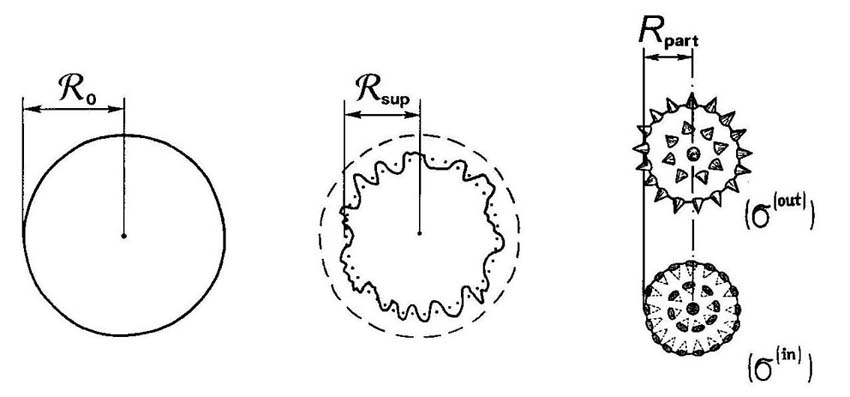Figure 5. The complete free primary ball, or superparticle (left), which being embedded in the tessellattice becomes crumpled (center), and the charge particle - two opposite polarized surface states correspond to the positive and negative electric charge (right) (from Ref. ). Thus the electric charge is kept and plays on the surface of the particle, which complete agrees with The Rigveda as has recently been decoded by Dr. R. R. M. Roy in his Vedic Physics. The magnetic charge has appeared at the motion of the particle: it is generated every odd half period λ/2 where λ is the amplitude of spatial oscillations of the particle, i.e. the particle's de Broglie wavelength. The magnetic charge is characterized by the bent state of needles on the surface of the particle .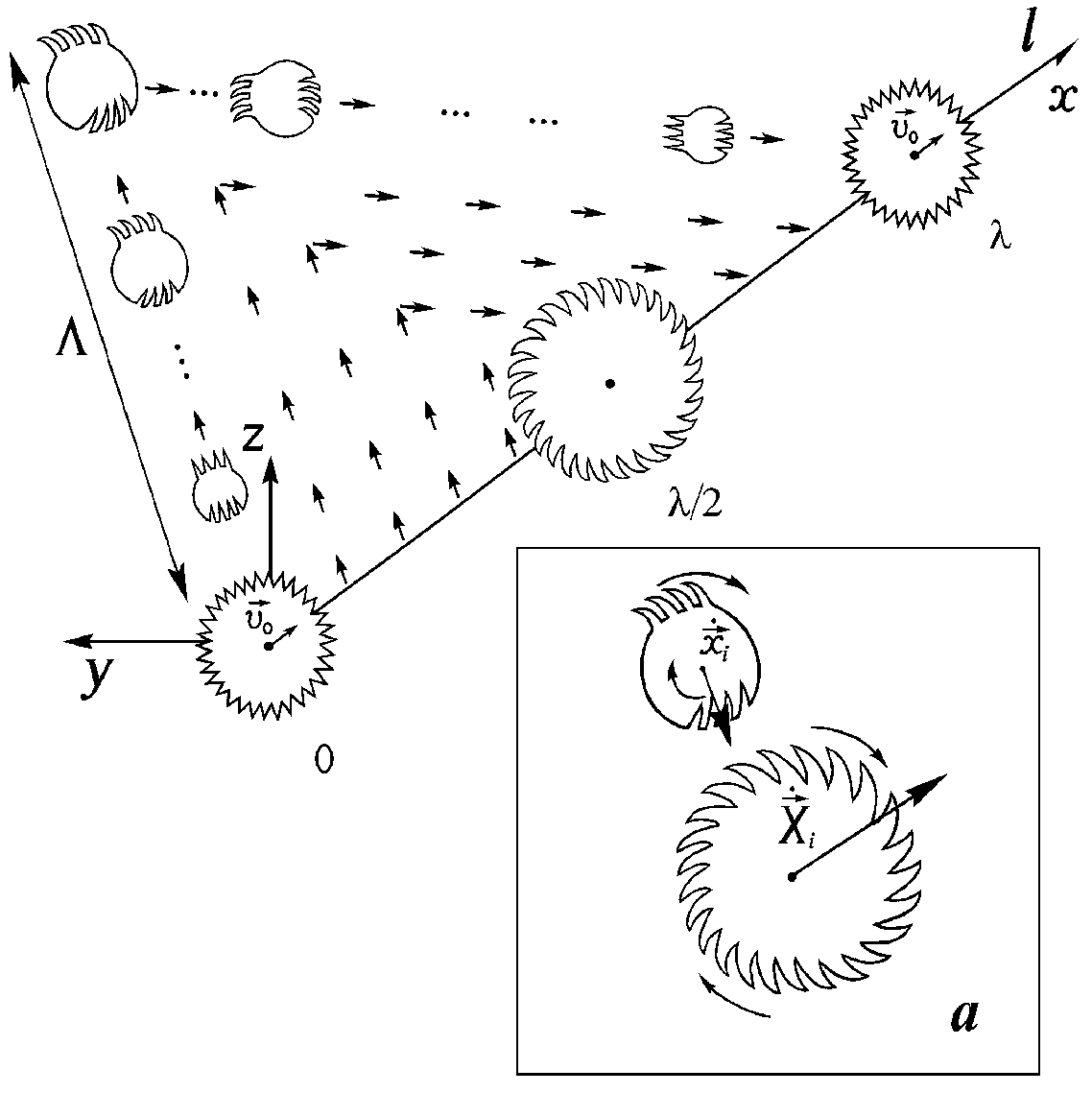Figure 6. Motion of the charged particle, for instance, the electron. The particle passing the half de Broglie wavelength λ/2 becomes the monopole, but to the end of the next section λ/2 the particle reverts to the initial pure electric state. Standing waves of inerton-photons, i.e. inertons polarized electromagnetically, accompany the particle (from Ref. ). How do a free inerton and a free photon look like? These excitations of the tessellattice migrate from cell to cell transferring their peculiar properties. The motion is characterized by periodicity, namely, the wavelength λ. The inerton carries fragments of cell's deformation: the deformed cell, i.e. mass m, is periodically transferred to the inflatied cell, the so-called antimass', which can be described by the oscillation of the radius of a cell between values R - Δr and R + Δr where R is the effective radius of a superparticle in the degenerate state, i.e. in the non-perturbed tessellattice.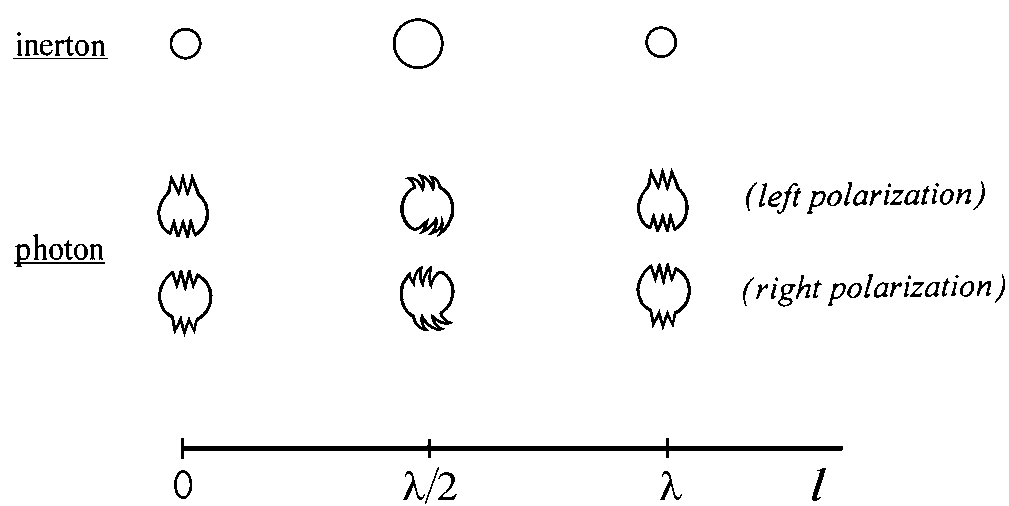Figure 7. Motion of two basic quasi-particles of the space: the free inerton and the free photon (from Ref. ). The photon transfers a surface polarization of a cell, which can have two possible orientations. During each spatial period, or wavelength λ, the electric polarizartion changes to the magnetic one. Experimental verification of the concept In paper  electrons moving in atoms were treated as entities surrounded by their inerton clouds. The investigation of the interaction between such extended entities and a photon flux was curried out in detail. The major peculiarity proposed was the effective cross-section of electrons significantly enlarged due to their inerton clouds spread around the electrons. A number of different experiments aimed at the study of laser-induced gas ionization were in agreement with the theoretical results prescribed by the inerton theory, though other theories failed to account for those data. The impact of the Earth inerton waves on the behavior of atoms in metals was studied theoretically and then observed experimentally in changes of the fine morphological structure of specimens by the high-resolution electron-scanning microscope . The inerton concept was applied  to explain a fine dynamics of hydrogen atoms in the crystal whose FTIR spectra in the 400 to 4000 cm-1 range showed unexplainable sub maximums. Features observed in the spectra were associated with the overlapping of hydrogen atoms' matter waves that induced the mean inerton field contributing to the paired potential of hydrogen-hydrogen interaction. Such additional interaction brought to the formation of hydrogen clusters that became the origin of the modulated spectra. The phenomenon of irradiation of aqueous solutions by scalar waves generated by the so-called Teslar® technology was studied in papers [25,26]. Our study justified that the Teslar watch produced nor the electromagnetic, neither ultrasound radiation. That was the inerton radiation generated by a special electric circuit embedded in the watch (two superimposed electromagnetic waves whose amplitudes are shifted to 180o are cancelled, but an inerton flow, which continues to transfer the energy, remains). These studies showed that the inerton field, in fact, carries a mass defect Δm, which is absorbed by entities in condensed media. In our experiment  laser illuminating ferroelectric crystal of LiNbO3 generated stable electron droplets contained around 1010 electrons, which freely moved with the velocity 0.5 cm/s in the air along the surface of the crystal experiencing the Earth gravitational field. We showed that the cluster stability was possible only due to the weighting of electrons up to millions of times, which happened due to the absorption of the crystal inertons by ejected electrons. Then in a cluster, electrons were interacting through two competitive potentials: the Coulomb one and the inerton field that a solitary one could elastically withstand the Coulomb repulsion. Consequently, the mass defect Δm becomes an inherent property not only of atomic nuclei but also of any physical and physical chemical systems. Here is the photos of our recent device that measures the inerton radiation (the intensity, i.e. how many inertons falls on 1 cm2 per 1 s and the frequency up to 100 kHz):Gravitation Gravity, as it is originated from the submicoscopic concept, macroscopic effects associated with the gravitation, and the explanation of well-known macroscopic experiments are shown in the appropriate papers [12,16,33,34,37,38] in the subsection Publications. Lyrical digression Consequently, just as from the viewpoint of conventional quantum physics classical theories are phenomenological, all modern quantum theories (quantum mechanics, electrodynamics, chromodynamics, etc.) in turn to be regarded as phenomenological from the developing submicroscopic standpoint. In other words, from a deeper submicroscopic understanding some interpretations and predictions of quantum theories are wrongly construed. No principal difficulties in the submicroscopic approach. However, there is a resistance, which rather very strong, from the editorial boards, but this is a typical situation for any new fundamental concept. Today physicists prefer to develop only formal methods, which are limited by predictions and deriving formulas of the stable states. Modern physical concepts do not take into consideration factors, which are responsible for fundamental notions researchers operate with every day. H. A. Lorentz and L. de Broglie intimated that the detailed description of physical phenomena - step-by-step - is also the goal of physics. Holding the idea of detailed description we should undoubtedly come to complete determinism that was strongly propagated by Henri Poincare, especially see his exceptionally bright last books: La valeur de la science, La science et l'hypothese, Science et methode and Dernieres pensees (Flammarion, Paris: 1905,1906, 1908 and 1913 respectively). Their position brings back G. Galilei's words: In questions of science, the authority of a thousand is not worth the humble reasoning of a single individual. In my study I attempt to recapture such an important avenue of investigation (see also Recent Events). RESEARCH INTERESTS Menu